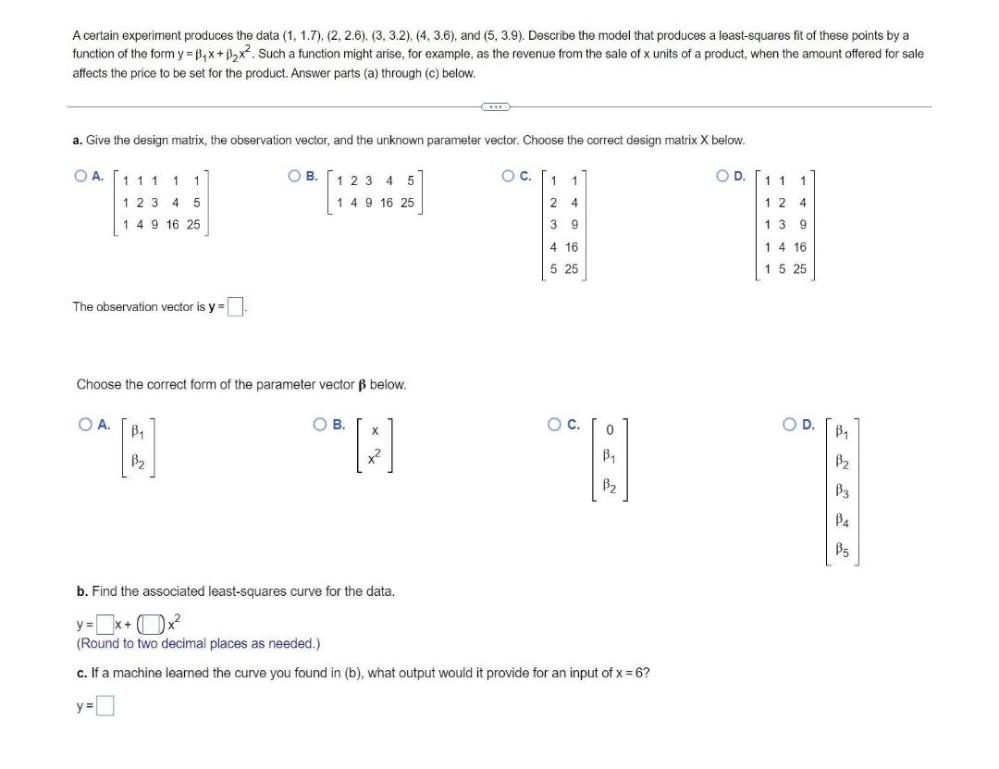Question:

# A certain experiment produces the data (1, 1.7), (2, 2.6), (3, 3.2), (4, 3.6), and (5, 3.9). Describe the model that produces a least-squares fit of these points by a function of the form y = β1*x+ β2A certain experiment produces the data (1, 1.7), (2, 2.6), (3, 3.2), (4, 3.6), and (5, 3.9). Describe the model that produces a least-squares fit of these points by a function of the form y = β1*x+ β2*x^2. Such a function might arise, for example, as the revenue from the sale of x units of a product, when the amount offered for sale affects the price to be set for the product. Answer parts (a) through (c) below. a. Give the design matrix, the observation vector, and the unknown parameter vector. Choose the correct design matrix X below. The observation vector is y = Choose the correct form of the parameter vector β below. b. Find the associated least-squares curve for the data. c. If a machine learned the curve you found in (b), what output would it provide for an input of x = 6?